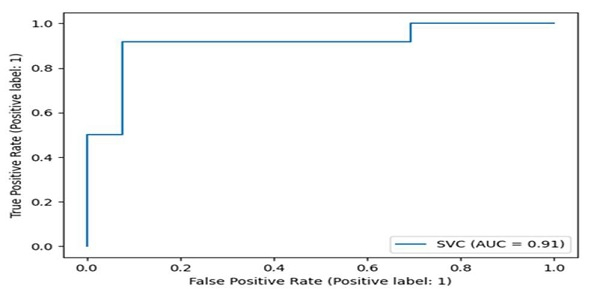# How to plot ROC curve in Python?

ROC − Receiver operating characteristics (ROC) curve.

Using metrics.plot_roc_curve(clf, X_test, y_test) method, we can draw the ROC curve.

## Steps

• Generate a random n-class classification problem. This initially creates clusters of points normally distributed (std=1) about vertices of an n_informative-dimensional hypercube with sides of length 2*class_sep and assigns an equal number of clusters to each class.

It introduces interdependence between these features and adds various types of further noise to the data. Use the make_classification() method.

• Split arrays or matrices into random trains, using train_test_split() method.

• Fit the SVM model according to the given training data, using fit() method.

• Plot Receiver operating characteristic (ROC) curve, using plot_roc_curve() method.

• To show the figure, use plt.show() method.

## Example

import matplotlib.pyplot as plt
from sklearn import datasets, metrics, model_selection, svm
X, y = datasets.make_classification(random_state=0)
X_train, X_test, y_train, y_test = model_selection.train_test_split(X, y, random_state=0)
clf = svm.SVC(random_state=0)
clf.fit(X_train, y_train)
metrics.plot_roc_curve(clf, X_test, y_test)
plt.show()

## OutputUpdated on: 16-Mar-2021

6K+ Views